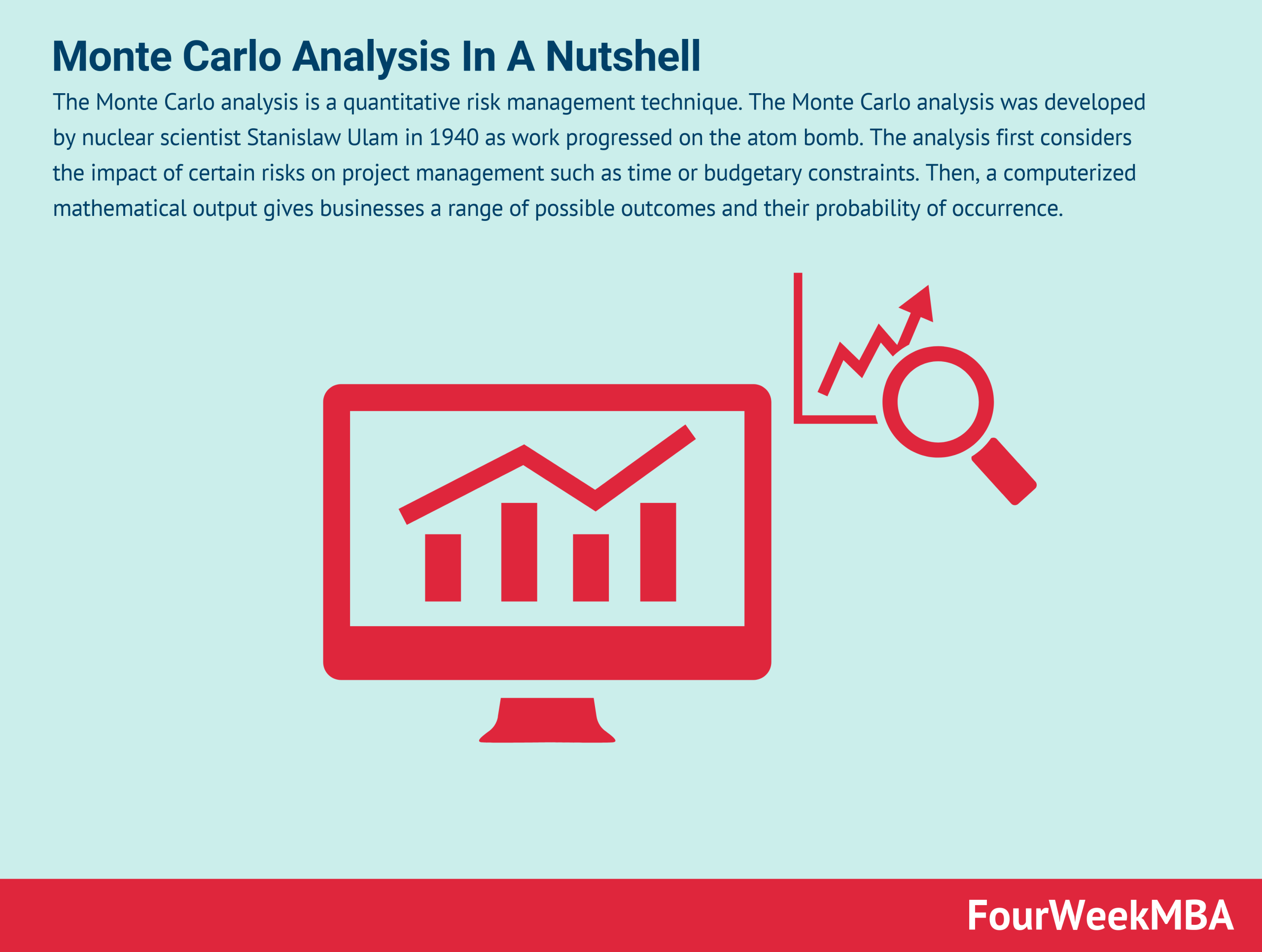# Monte Carlo Analysis In A Nutshell

The Monte Carlo analysis is a quantitative risk management technique. The Monte Carlo analysis was developed by nuclear scientist Stanislaw Ulam in 1940 as work progressed on the atom bomb. The analysis first considers the impact of certain risks on project management such as time or budgetary constraints. Then, a computerized mathematical output gives businesses a range of possible outcomes and their probability of occurrence.

## Understanding the Monte Carlo analysis

The analysis first considers the impact of certain risks on project management such as time or budgetary constraints. Then, a computerized mathematical output gives businesses a range of possible outcomes and their probability of occurrence. The output shows the potential consequences for the most and least conservative actions and details the middle-of-the-road actions that fall in between.

Probability distributions allow businesses to quantitatively determine the level of risk associated with decision making. In turn, the decision with the most optimal balance of benefit and risk can be selected.

The Monte Carlo analysis is used in a broad swathe of industries such as finance, manufacturing, insurance, and transportation.

## Conducting a Monte Carlo analysis

The first requirement of a Monte Carlo analysis is spreadsheet data. Most spreadsheets incorporate:

• Outputs – such as cash flow, profit, or sales volume.
• Inputs – or quantitative factors such as market size, material cost, or production capacity.

For example, a company that builds prefabricated homes might have output data on the total cost of building each home. Input data would quantify the cost of each component, such as the foundation, plastering, windows, and land acquisition. For each input, the company then determines a minimum, maximum, and best guess value.

This is performed because component costs tend to fluctuate. By establishing a minimum and maximum value for each input cost, the business has an idea of the uncertainty of the total output value. The best guess value also determines what the project is likely to cost.

However, there is a better way to calculate uncertainty.

### The power of computers

The simple spreadsheet analysis that the home construction company uses has several drawbacks. It does not consider probabilities of a scenario, nor does it consider the number of combinations that could constitute a scenario. Indeed, if the company uses 11 input variables with each valued three different ways, over 177,000 combinations can influence uncertainty.

The Monte Carlo analysis replaces the simple “three valuemodel with complex functions that generate random samples. These random samples are represented by probability distributions that represent uncertainty in a vast number of scenarios.

## Benefits of the Monte Carlo analysis

The primary benefit of the Monte Carlo analysis lies in moving uncertainty from a single simulation to a probabilistic simulation.

Returning to the home construction company:

• A single simulation of an uncertain system is usually a qualified statement. For example, “If the cost of cement reaches a certain price, our business model may become unprofitable.”
• The result of a probabilistic Monte Carlo analysis is a quantifiable probability. For example, “If the cost of cement reaches a certain price, there is a 35% chance that our business model becomes unprofitable.

As we have seen, there is also an inherent benefit in the computational power of complex data analysis

The Monte Carlo analysis provides many separate and independent results, with each suggesting a possible future scenario. Results are attained quickly and accurately using common probability distributions such as normal, lognormal, uniform, and triangular.

Ultimately, probability distributions are a much more realistic way of describing variable uncertainty in risk analysis. This helps businesses prepare for and manage risk.

## Key takeaways

• The Monte Carlo analysis is a risk management technique that uses probability distributions.
• The Monte Carlo analysis allows decision-makers to determine the level of risk in making each decision. The analysis uses mathematical functions to generate many thousands of sample scenarios based on the complex interaction of input values and variables.
• The Monte Carlo analysis helps businesses move away from simplistic risk assessment decisions by using powerful computational methods that yield fast and accurate results.

Main Guides:

Main Case Studies:

Scroll to Top
[class^="wpforms-"]
[class^="wpforms-"]